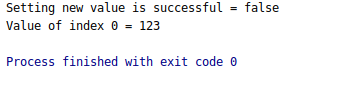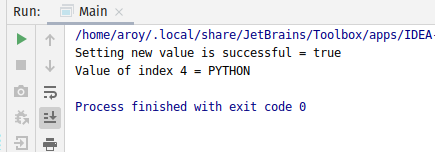# AtomicReferenceArray weakCompareAndSetPlain() method in Java with Examples

• Last Updated : 03 Jan, 2020

The weakCompareAndSetPlain() method of a AtomicReferenceArray class is used to atomically sets the value of the element at index i to newValue to newValue for AtomicReferenceArray if the current value is equal to expectedValue passed as parameter. This method updates the value at index i with memory semantics of setting as if the variable at index i was declared non-volatile and non-final.This method returns true if set a new value is successful.

Syntax:

```public final boolean weakCompareAndSetPlain(int i,
E expectedValue,
E newValue)
```

Parameters: This method accepts i which is an index of AtomicReferenceArray to perform the operation, expectedValue which is the expected value and newValue which is the new value to set.

Return value: This method returns true if successful.

Below programs illustrate the weakCompareAndSetPlain() method:
Program 1:

 `// Java program to demonstrate AtomicReferenceArray``// weakCompareAndSetPlain() method`` ` `import` `java.util.concurrent.atomic.AtomicReferenceArray;`` ` `public` `class` `GFG {`` ` `    ``public` `static` `void` `main(String[] args)``    ``{`` ` `        ``// create an atomic reference object.``        ``AtomicReferenceArray ref``            ``= ``new` `AtomicReferenceArray(``5``);`` ` `        ``// set some value``        ``ref.set(``0``, ``321``);``        ``ref.set(``1``, ``123``);``        ``ref.set(``2``, ``322``);`` ` `        ``// apply weakCompareAndSetPlain()``        ``boolean` `result``            ``= ref.weakCompareAndSetPlain(``                ``0``, ``124``,``                ``234``);`` ` `        ``// print value``        ``System.out.println(``"Setting new value"``                           ``+ ``" is successful = "``                           ``+ result);`` ` `        ``System.out.println(``"Value of index 0 = "``                           ``+ ref.get(``1``));``    ``}``}`

Output:Program 2:

 `// Java program to demonstrate AtomicReferenceArray``// weakCompareAndSetPlain() method`` ` `import` `java.util.concurrent.atomic.AtomicReferenceArray;`` ` `public` `class` `GFG {`` ` `    ``public` `static` `void` `main(String[] args)``    ``{`` ` `        ``// create an atomic reference object.c``        ``AtomicReferenceArray ref``            ``= ``new` `AtomicReferenceArray(``5``);`` ` `        ``// set some value``        ``ref.set(``0``, ``"C"``);``        ``ref.set(``1``, ``"JAVA"``);``        ``ref.set(``2``, ``"JS"``);``        ``ref.set(``3``, ``"C++"``);``        ``ref.set(``4``, ``"C"``);`` ` `        ``// apply weakCompareAndSetVolatile()``        ``boolean` `result``            ``= ref.weakCompareAndSetVolatile(``                ``4``, ``"C"``,``                ``"PYTHON"``);`` ` `        ``// print value``        ``System.out.println(``"Setting new value"``                           ``+ ``" is successful = "``                           ``+ result);`` ` `        ``System.out.println(``"Value of index 4 = "``                           ``+ ref.get(``4``));``    ``}``}`

Output:My Personal Notes arrow_drop_up